Скачать презентацию Conic Sections Mathworld Circle National Science Foundation

6729175bfdec7c53fe677297846eff8a.ppt

• Количество слайдов: 58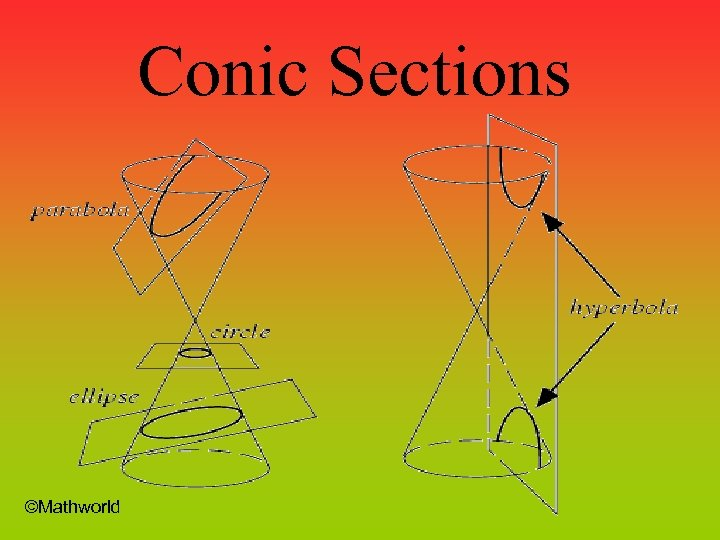Conic Sections ©Mathworld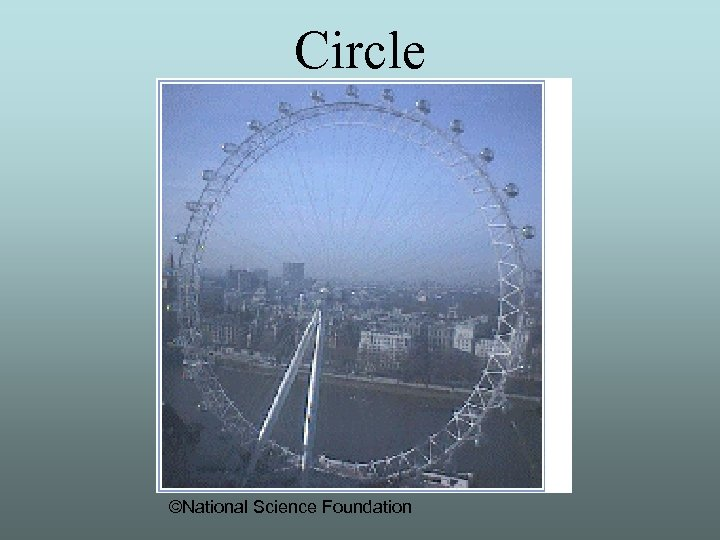Circle ©National Science Foundation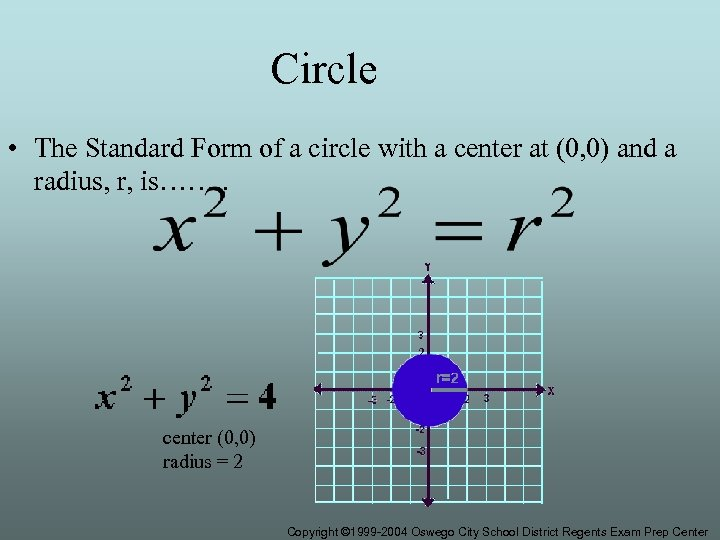Circle • The Standard Form of a circle with a center at (0, 0) and a radius, r, is……. . center (0, 0) radius = 2 Copyright © 1999 -2004 Oswego City School District Regents Exam Prep Center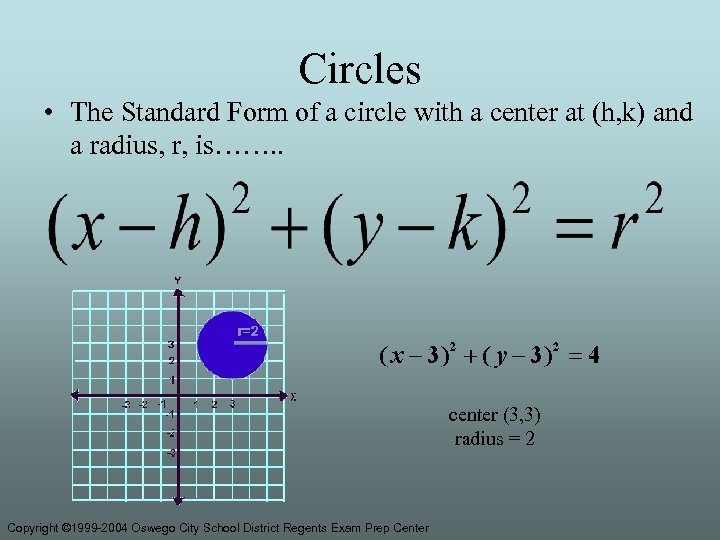Circles • The Standard Form of a circle with a center at (h, k) and a radius, r, is……. . center (3, 3) radius = 2 Copyright © 1999 -2004 Oswego City School District Regents Exam Prep Center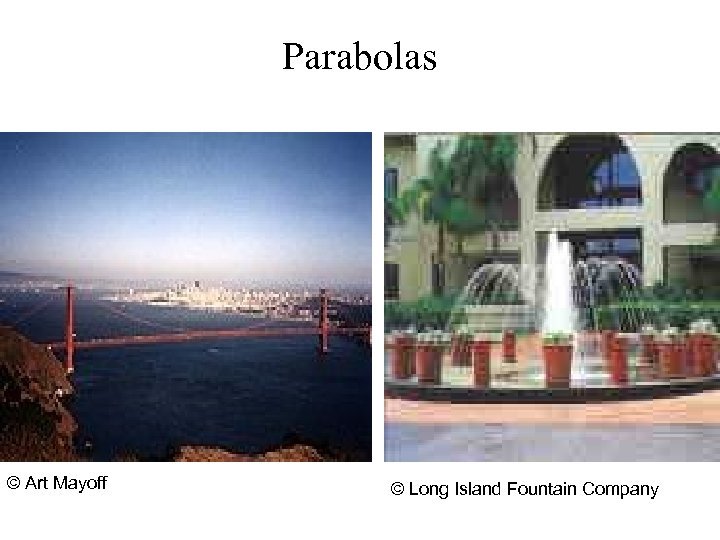Parabolas © Art Mayoff © Long Island Fountain Company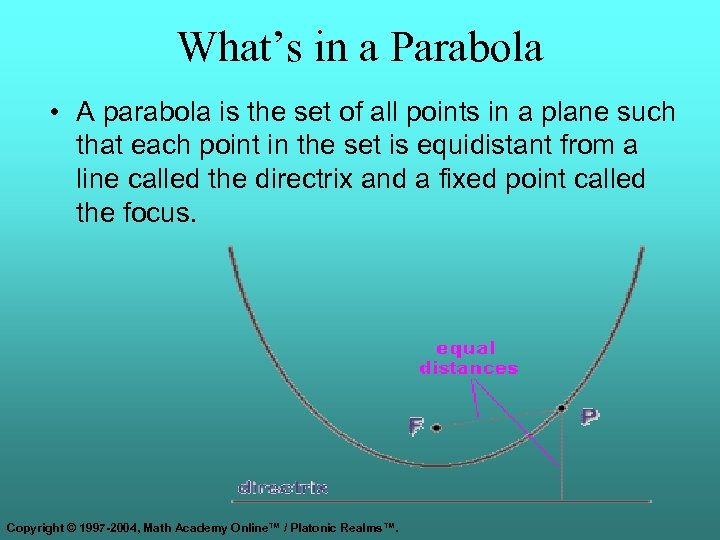What’s in a Parabola • A parabola is the set of all points in a plane such that each point in the set is equidistant from a line called the directrix and a fixed point called the focus. Copyright © 1997 -2004, Math Academy Online™ / Platonic Realms™.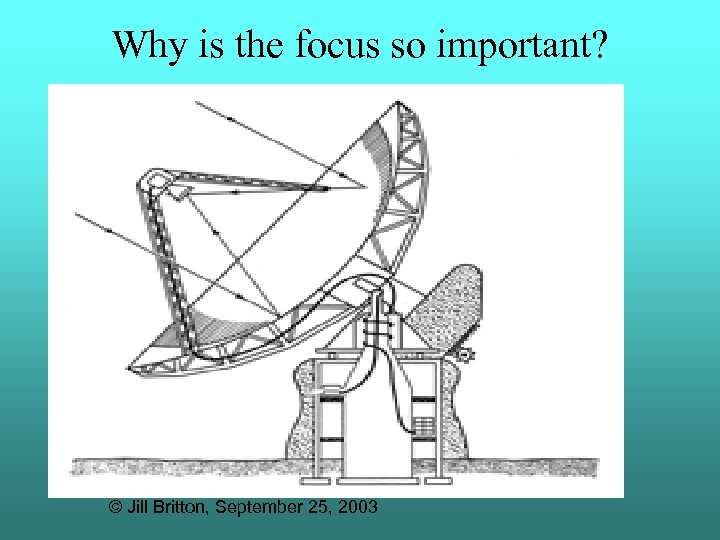Why is the focus so important? © Jill Britton, September 25, 2003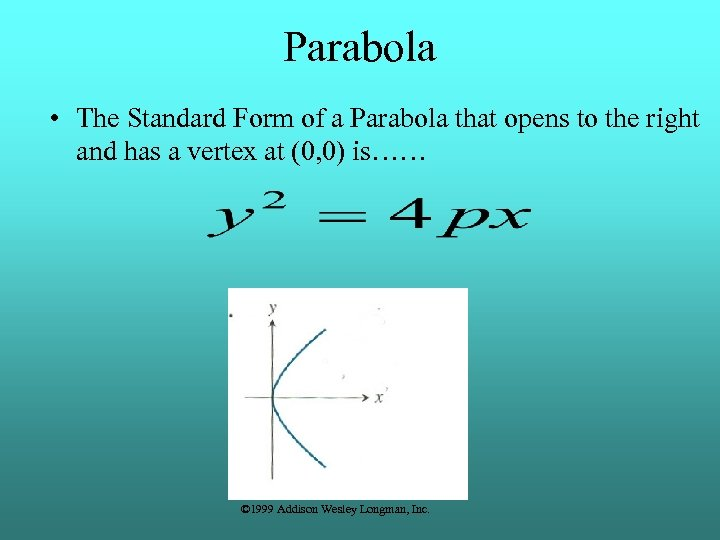Parabola • The Standard Form of a Parabola that opens to the right and has a vertex at (0, 0) is…… © 1999 Addison Wesley Longman, Inc.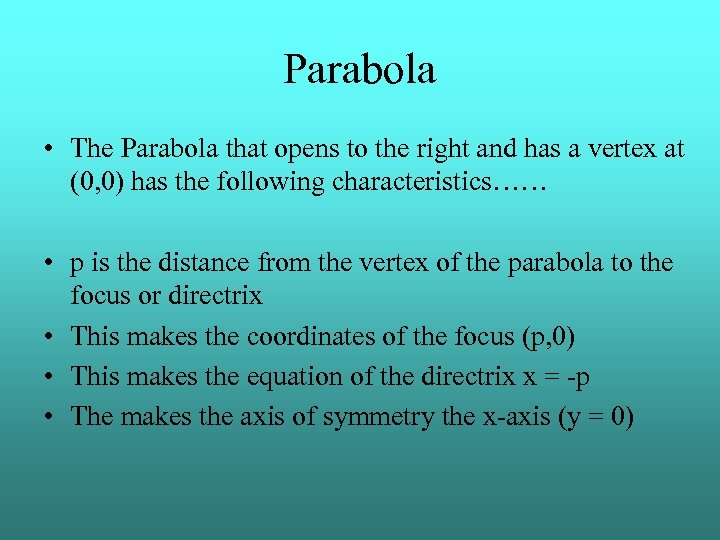Parabola • The Parabola that opens to the right and has a vertex at (0, 0) has the following characteristics…… • p is the distance from the vertex of the parabola to the focus or directrix • This makes the coordinates of the focus (p, 0) • This makes the equation of the directrix x = -p • The makes the axis of symmetry the x-axis (y = 0)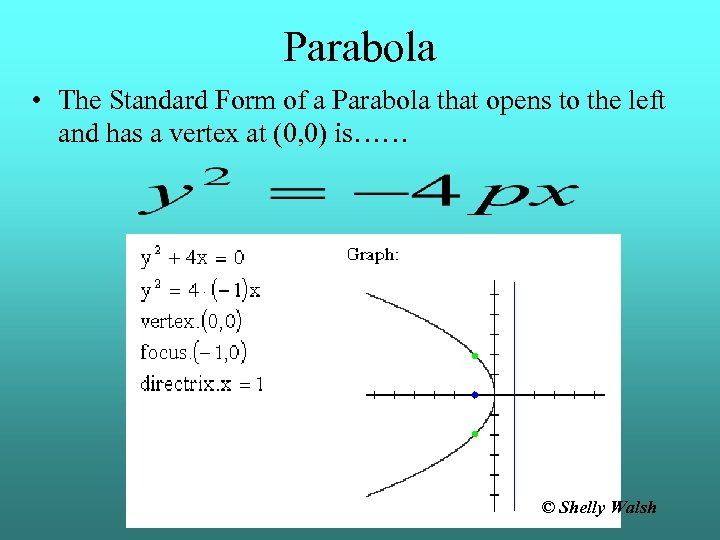Parabola • The Standard Form of a Parabola that opens to the left and has a vertex at (0, 0) is…… © Shelly Walsh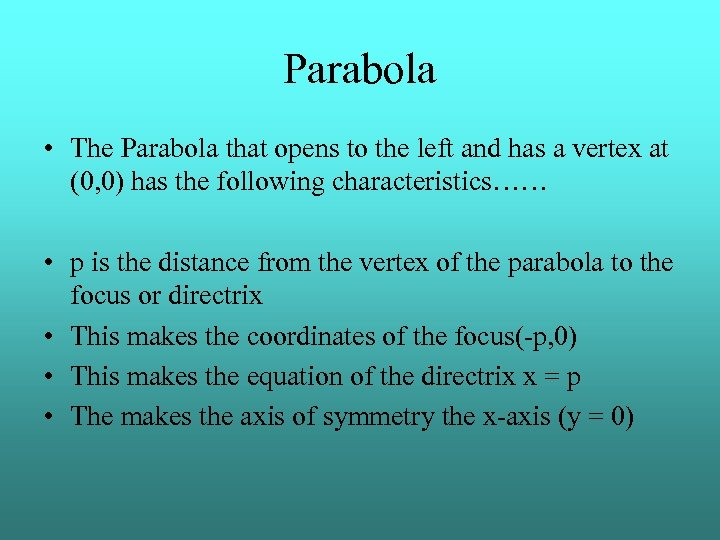Parabola • The Parabola that opens to the left and has a vertex at (0, 0) has the following characteristics…… • p is the distance from the vertex of the parabola to the focus or directrix • This makes the coordinates of the focus(-p, 0) • This makes the equation of the directrix x = p • The makes the axis of symmetry the x-axis (y = 0)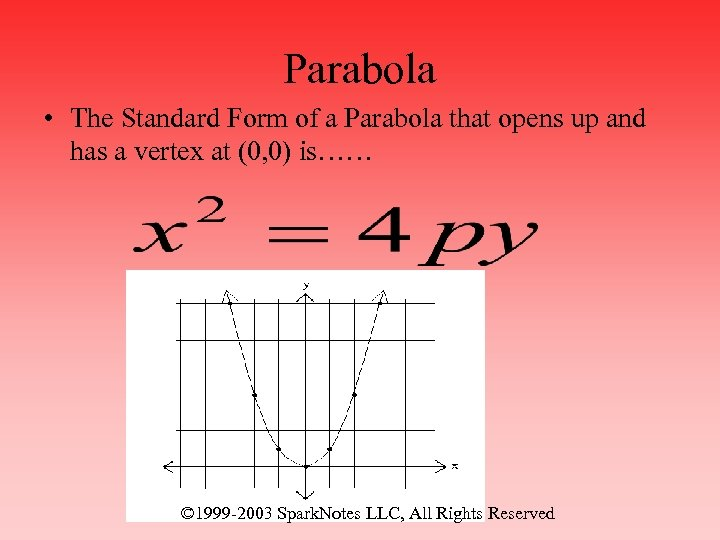Parabola • The Standard Form of a Parabola that opens up and has a vertex at (0, 0) is…… © 1999 -2003 Spark. Notes LLC, All Rights Reserved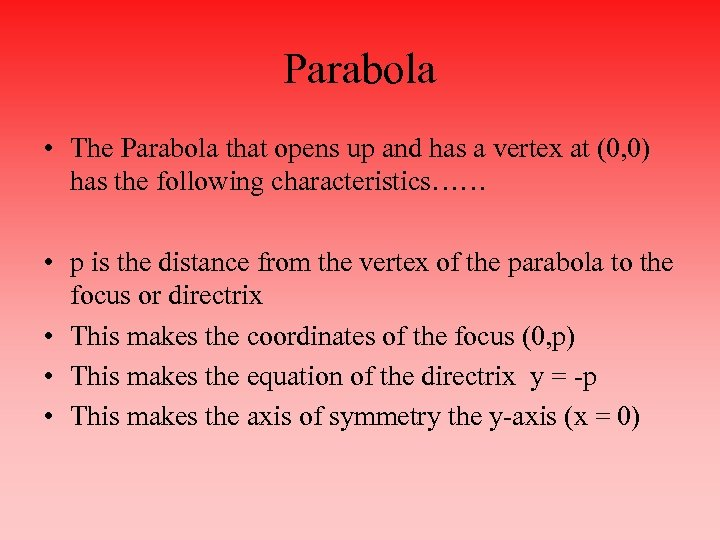Parabola • The Parabola that opens up and has a vertex at (0, 0) has the following characteristics…… • p is the distance from the vertex of the parabola to the focus or directrix • This makes the coordinates of the focus (0, p) • This makes the equation of the directrix y = -p • This makes the axis of symmetry the y-axis (x = 0)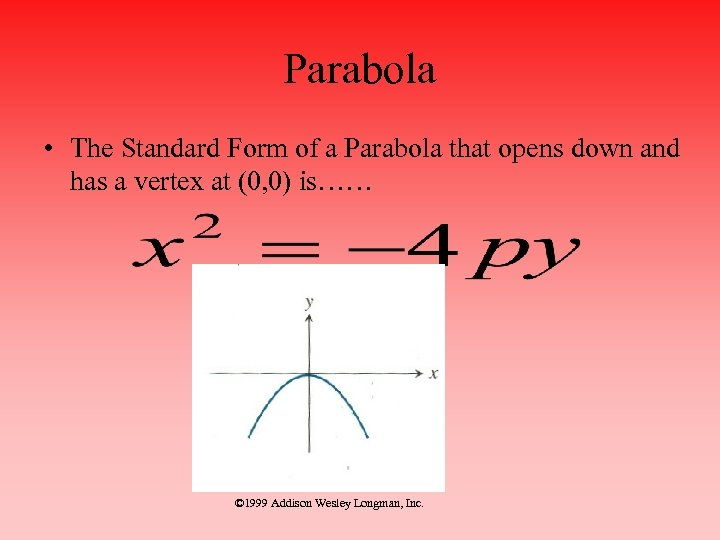Parabola • The Standard Form of a Parabola that opens down and has a vertex at (0, 0) is…… © 1999 Addison Wesley Longman, Inc.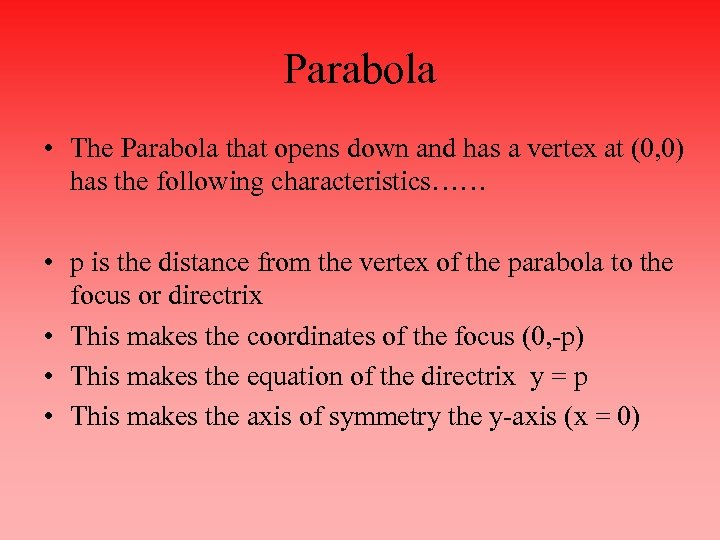Parabola • The Parabola that opens down and has a vertex at (0, 0) has the following characteristics…… • p is the distance from the vertex of the parabola to the focus or directrix • This makes the coordinates of the focus (0, -p) • This makes the equation of the directrix y = p • This makes the axis of symmetry the y-axis (x = 0)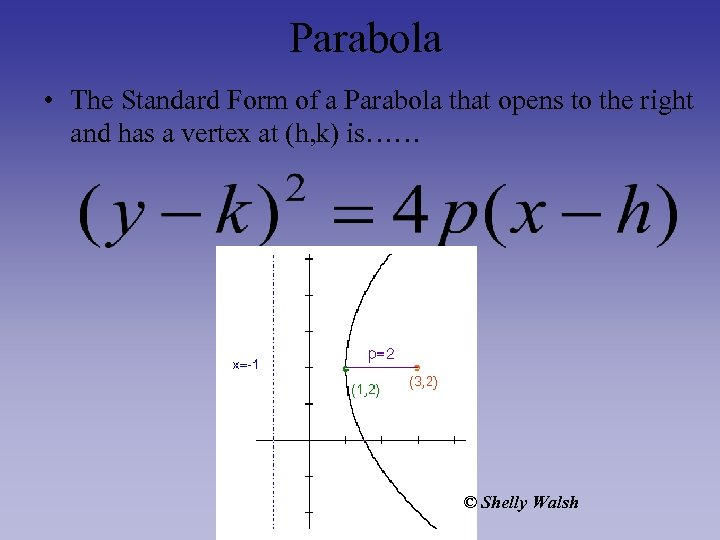Parabola • The Standard Form of a Parabola that opens to the right and has a vertex at (h, k) is…… © Shelly Walsh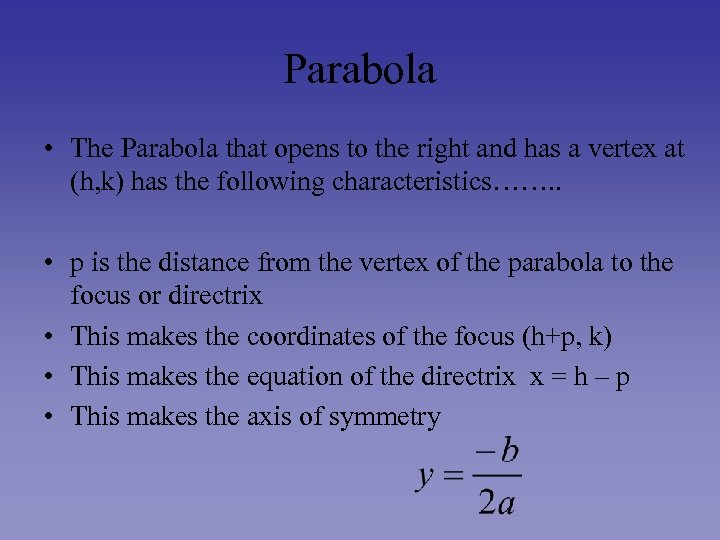Parabola • The Parabola that opens to the right and has a vertex at (h, k) has the following characteristics……. . • p is the distance from the vertex of the parabola to the focus or directrix • This makes the coordinates of the focus (h+p, k) • This makes the equation of the directrix x = h – p • This makes the axis of symmetry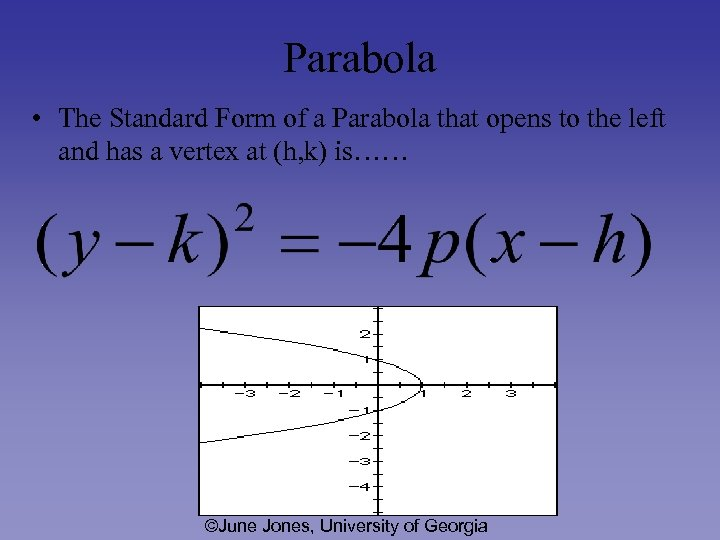Parabola • The Standard Form of a Parabola that opens to the left and has a vertex at (h, k) is…… ©June Jones, University of Georgia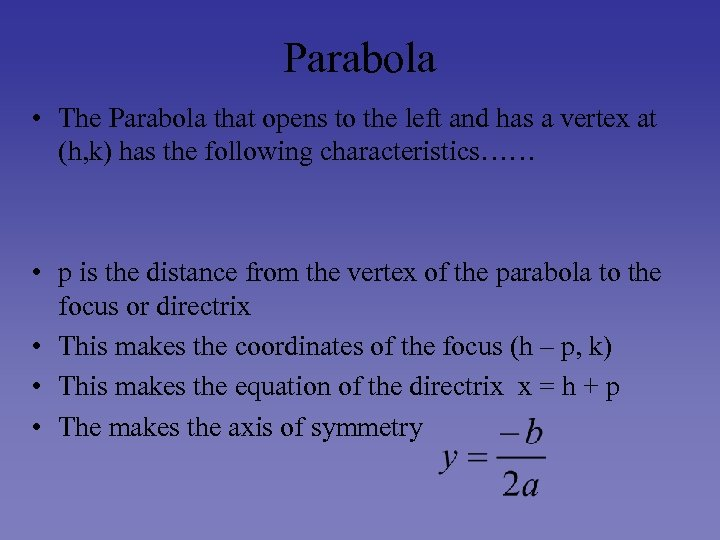Parabola • The Parabola that opens to the left and has a vertex at (h, k) has the following characteristics…… • p is the distance from the vertex of the parabola to the focus or directrix • This makes the coordinates of the focus (h – p, k) • This makes the equation of the directrix x = h + p • The makes the axis of symmetry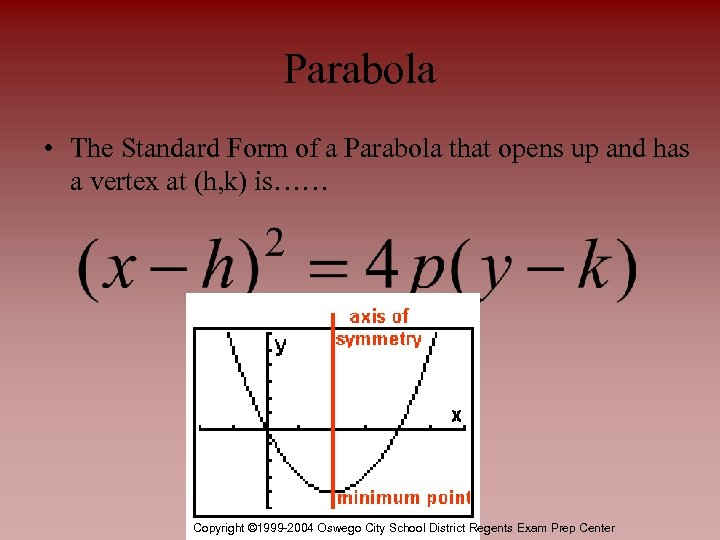Parabola • The Standard Form of a Parabola that opens up and has a vertex at (h, k) is…… Copyright © 1999 -2004 Oswego City School District Regents Exam Prep Center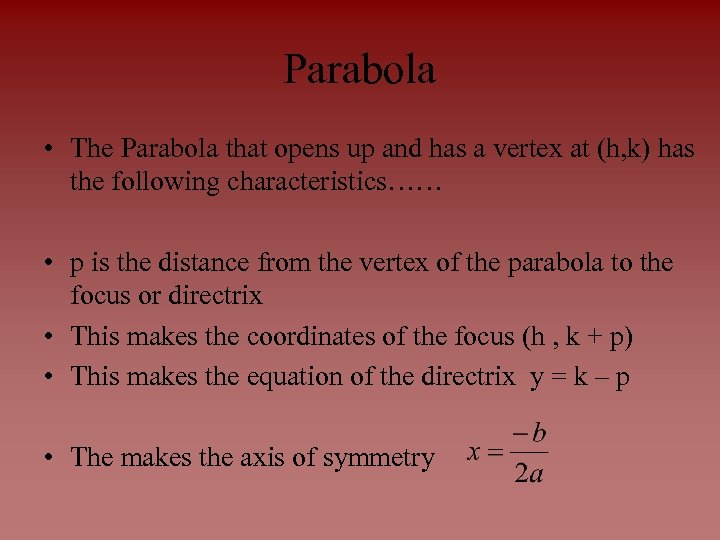Parabola • The Parabola that opens up and has a vertex at (h, k) has the following characteristics…… • p is the distance from the vertex of the parabola to the focus or directrix • This makes the coordinates of the focus (h , k + p) • This makes the equation of the directrix y = k – p • The makes the axis of symmetry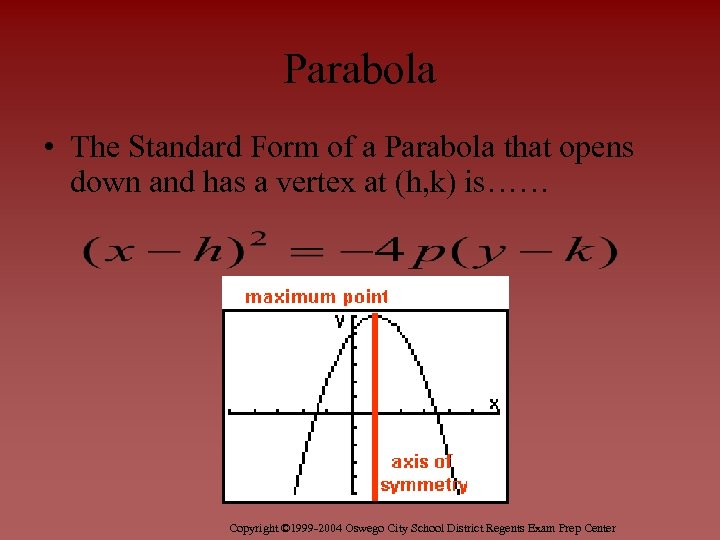Parabola • The Standard Form of a Parabola that opens down and has a vertex at (h, k) is…… Copyright © 1999 -2004 Oswego City School District Regents Exam Prep Center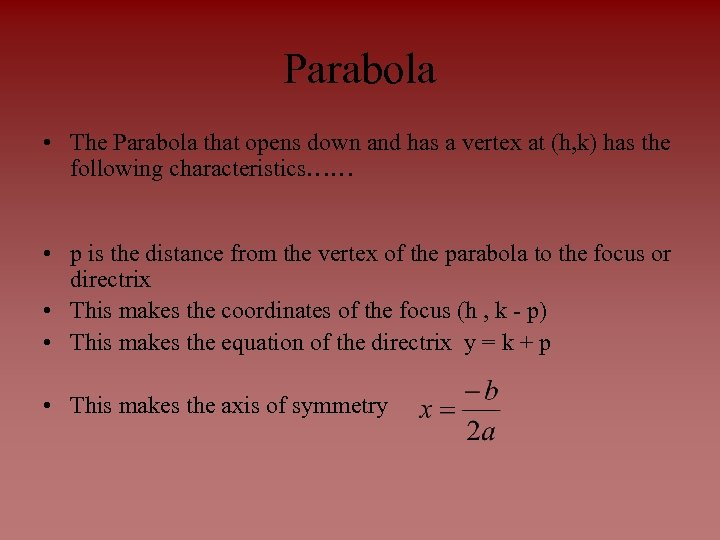Parabola • The Parabola that opens down and has a vertex at (h, k) has the following characteristics…… • p is the distance from the vertex of the parabola to the focus or directrix • This makes the coordinates of the focus (h , k - p) • This makes the equation of the directrix y = k + p • This makes the axis of symmetry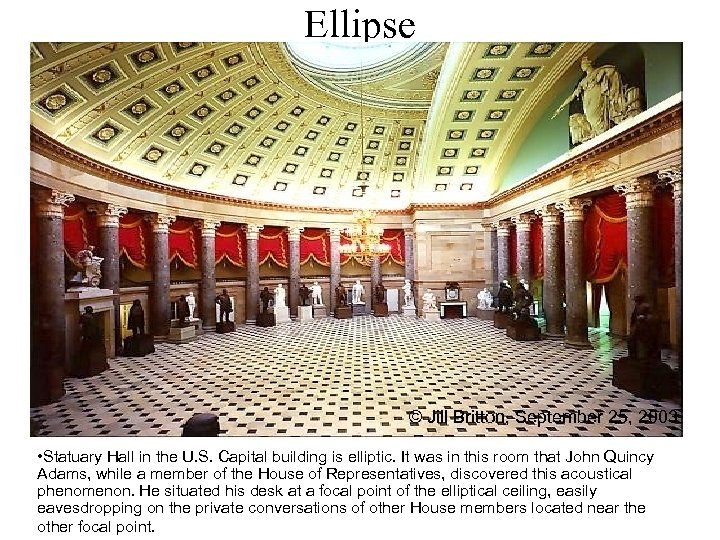Ellipse © Jill Britton, September 25, 2003 • Statuary Hall in the U. S. Capital building is elliptic. It was in this room that John Quincy Adams, while a member of the House of Representatives, discovered this acoustical phenomenon. He situated his desk at a focal point of the elliptical ceiling, easily eavesdropping on the private conversations of other House members located near the other focal point.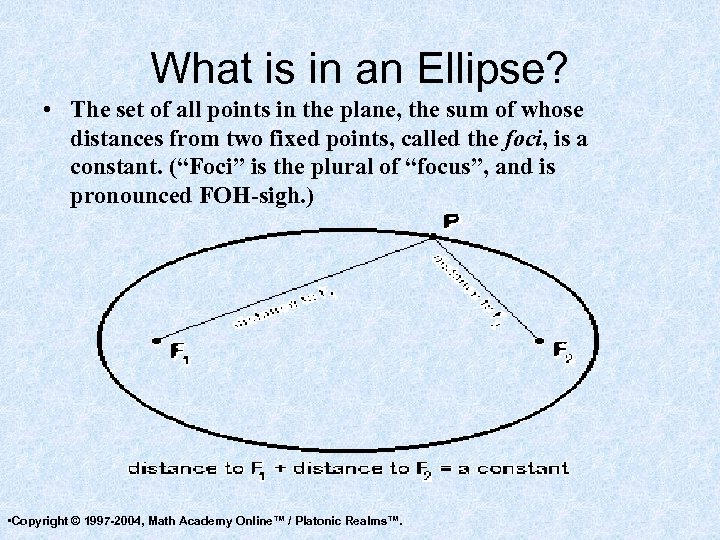What is in an Ellipse? • The set of all points in the plane, the sum of whose distances from two fixed points, called the foci, is a constant. (“Foci” is the plural of “focus”, and is pronounced FOH-sigh. ) • Copyright © 1997 -2004, Math Academy Online™ / Platonic Realms™.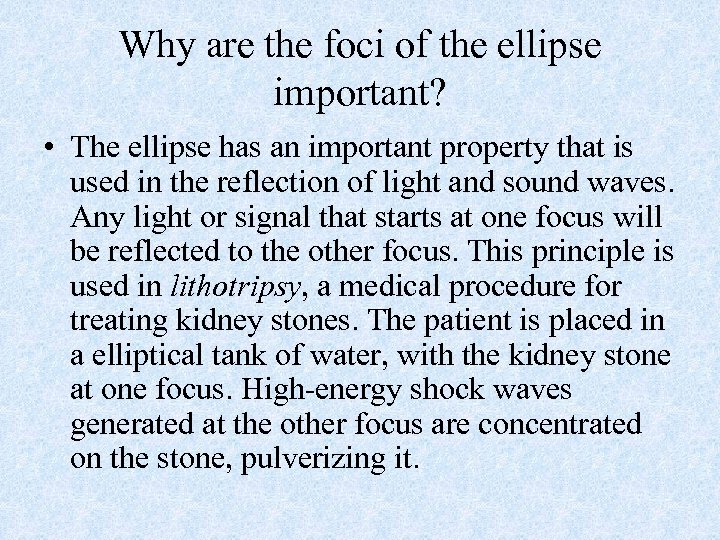Why are the foci of the ellipse important? • The ellipse has an important property that is used in the reflection of light and sound waves. Any light or signal that starts at one focus will be reflected to the other focus. This principle is used in lithotripsy, a medical procedure for treating kidney stones. The patient is placed in a elliptical tank of water, with the kidney stone at one focus. High-energy shock waves generated at the other focus are concentrated on the stone, pulverizing it.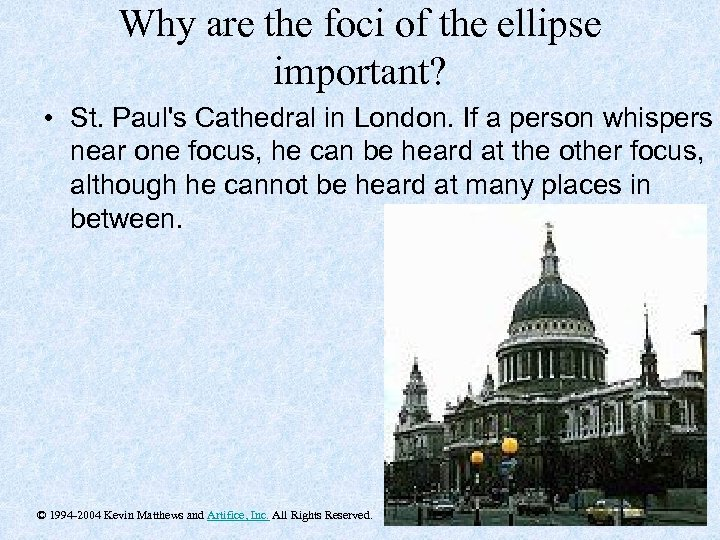Why are the foci of the ellipse important? • St. Paul's Cathedral in London. If a person whispers near one focus, he can be heard at the other focus, although he cannot be heard at many places in between. © 1994 -2004 Kevin Matthews and Artifice, Inc. All Rights Reserved.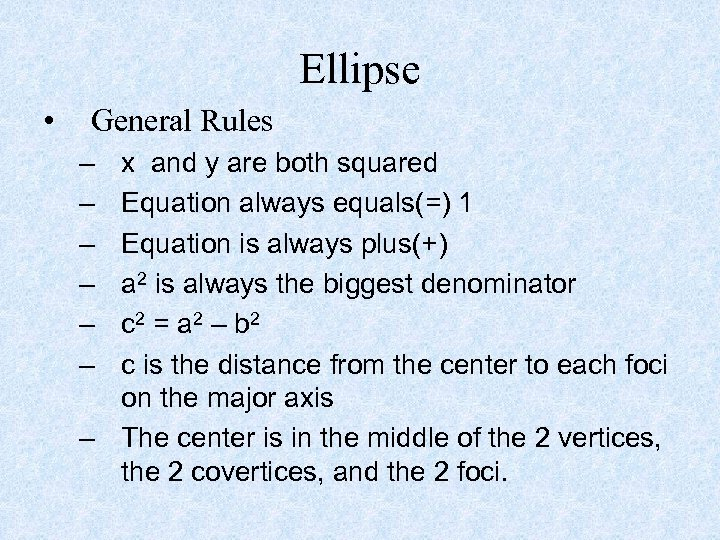Ellipse • General Rules – – – x and y are both squared Equation always equals(=) 1 Equation is always plus(+) a 2 is always the biggest denominator c 2 = a 2 – b 2 c is the distance from the center to each foci on the major axis – The center is in the middle of the 2 vertices, the 2 covertices, and the 2 foci.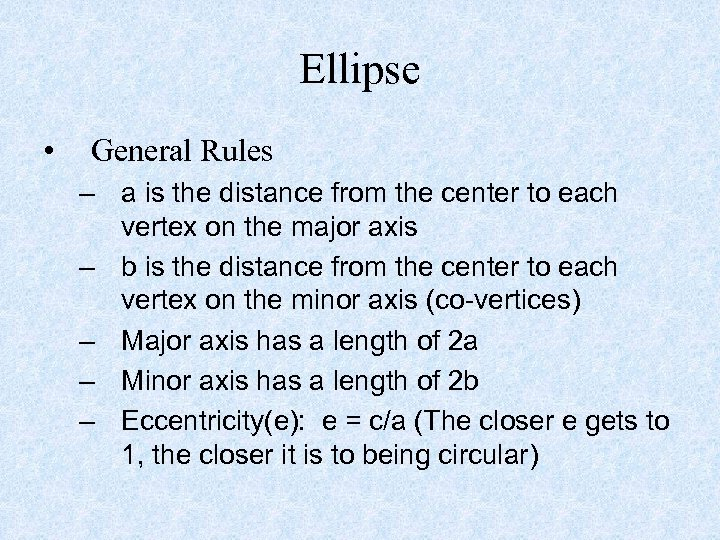Ellipse • General Rules – a is the distance from the center to each vertex on the major axis – b is the distance from the center to each vertex on the minor axis (co-vertices) – Major axis has a length of 2 a – Minor axis has a length of 2 b – Eccentricity(e): e = c/a (The closer e gets to 1, the closer it is to being circular)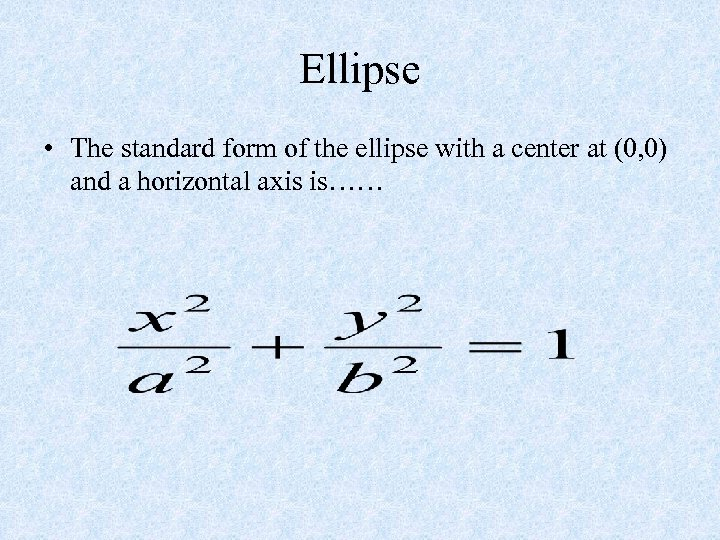Ellipse • The standard form of the ellipse with a center at (0, 0) and a horizontal axis is……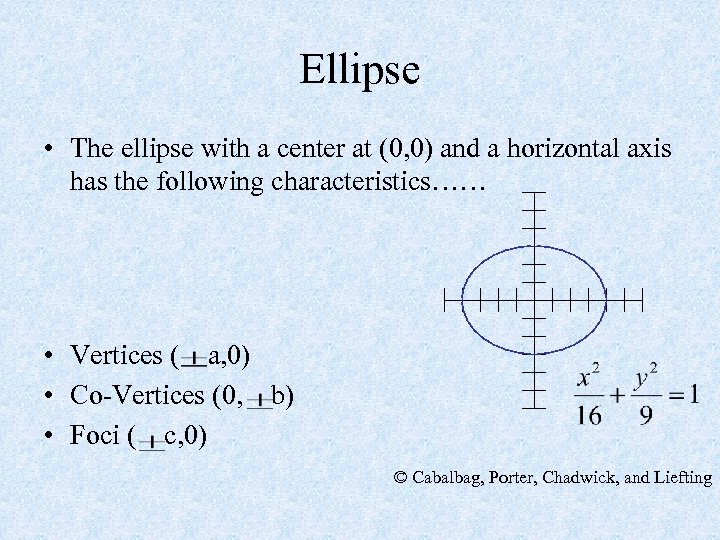Ellipse • The ellipse with a center at (0, 0) and a horizontal axis has the following characteristics…… • Vertices ( a, 0) • Co-Vertices (0, b) • Foci ( c, 0) © Cabalbag, Porter, Chadwick, and Liefting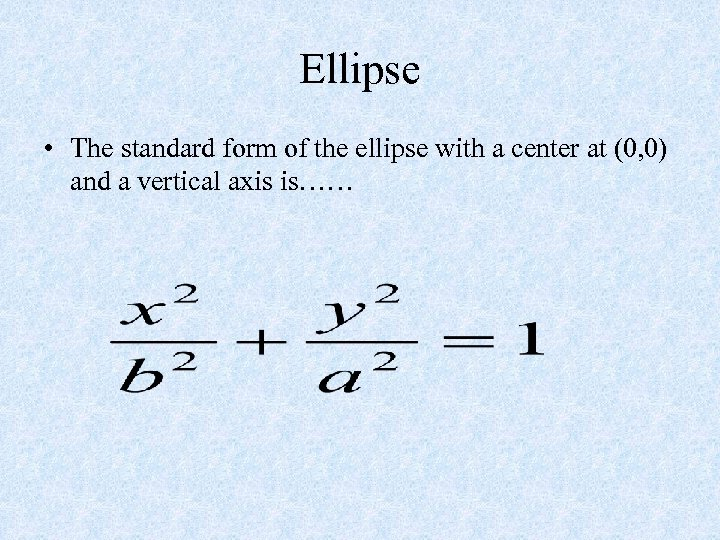Ellipse • The standard form of the ellipse with a center at (0, 0) and a vertical axis is……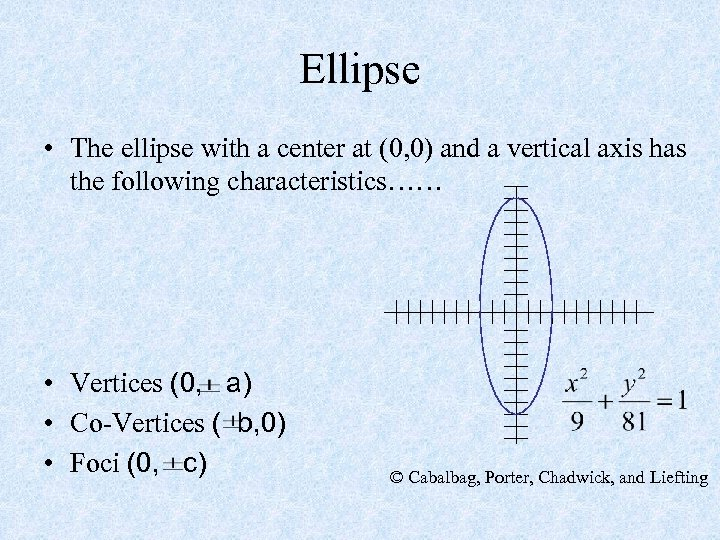Ellipse • The ellipse with a center at (0, 0) and a vertical axis has the following characteristics…… • Vertices (0, a) • Co-Vertices ( b, 0) • Foci (0, c) © Cabalbag, Porter, Chadwick, and Liefting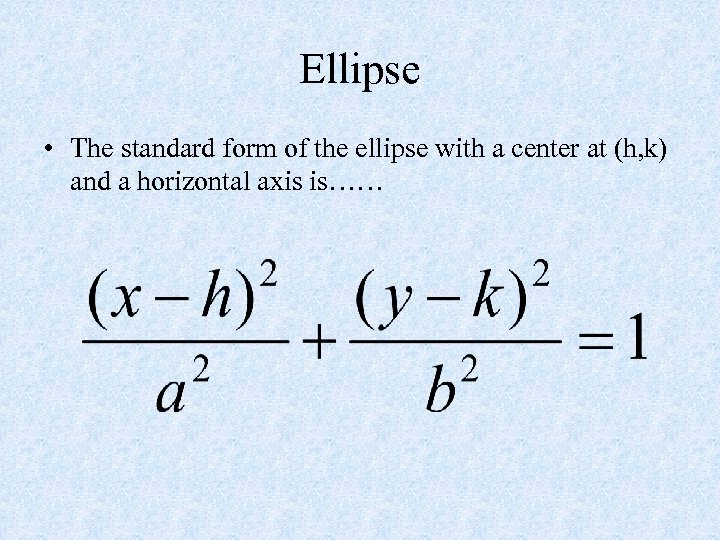Ellipse • The standard form of the ellipse with a center at (h, k) and a horizontal axis is……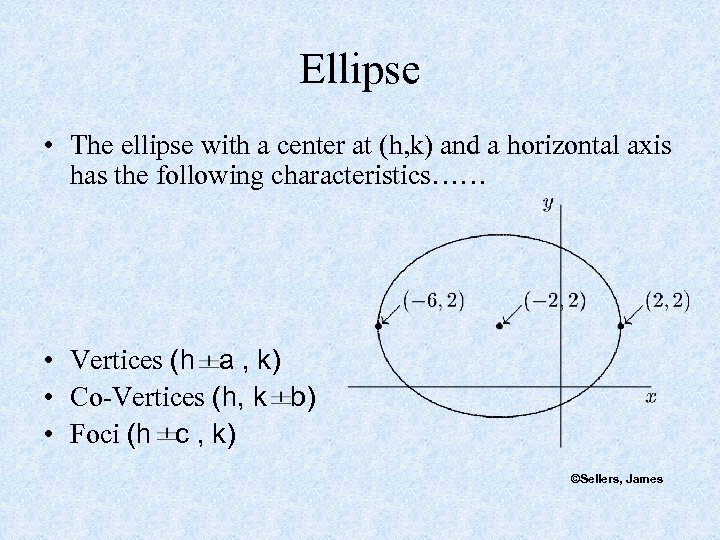Ellipse • The ellipse with a center at (h, k) and a horizontal axis has the following characteristics…… • Vertices (h a , k) • Co-Vertices (h, k b) • Foci (h c , k) ©Sellers, James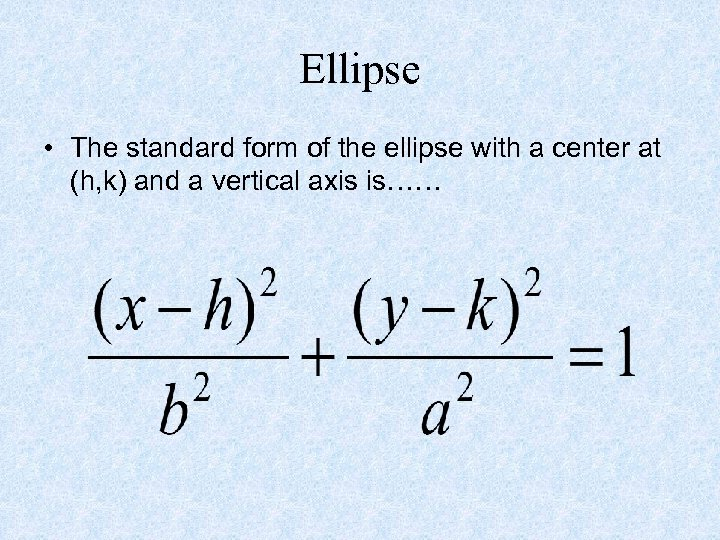Ellipse • The standard form of the ellipse with a center at (h, k) and a vertical axis is……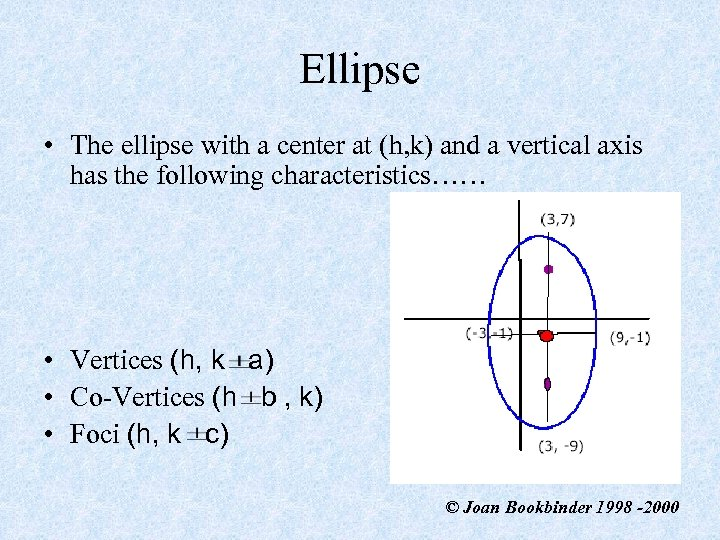Ellipse • The ellipse with a center at (h, k) and a vertical axis has the following characteristics…… • Vertices (h, k a) • Co-Vertices (h b , k) • Foci (h, k c) © Joan Bookbinder 1998 -2000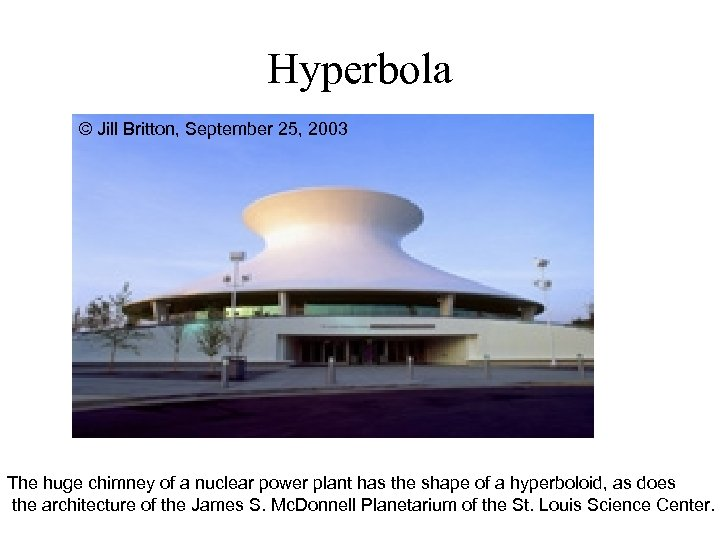Hyperbola © Jill Britton, September 25, 2003 The huge chimney of a nuclear power plant has the shape of a hyperboloid, as does the architecture of the James S. Mc. Donnell Planetarium of the St. Louis Science Center.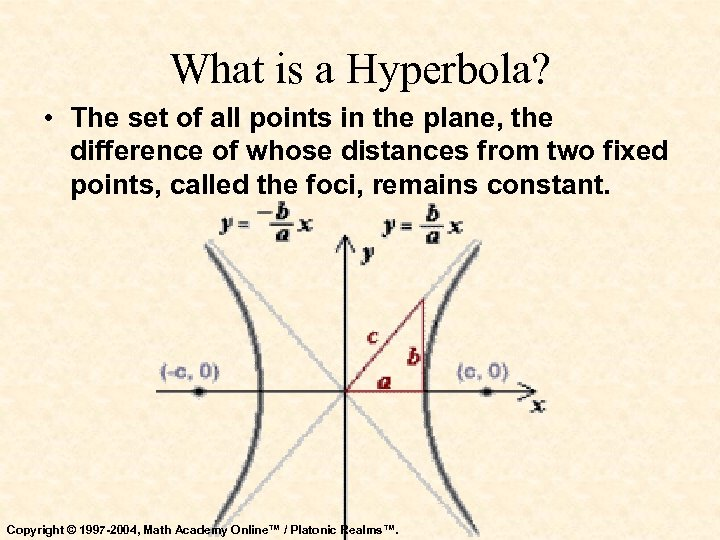What is a Hyperbola? • The set of all points in the plane, the difference of whose distances from two fixed points, called the foci, remains constant. Copyright © 1997 -2004, Math Academy Online™ / Platonic Realms™.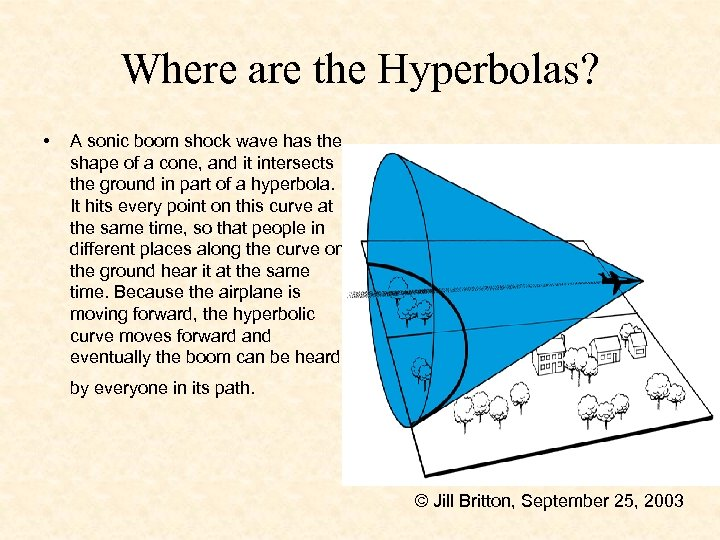Where are the Hyperbolas? • A sonic boom shock wave has the shape of a cone, and it intersects the ground in part of a hyperbola. It hits every point on this curve at the same time, so that people in different places along the curve on the ground hear it at the same time. Because the airplane is moving forward, the hyperbolic curve moves forward and eventually the boom can be heard by everyone in its path. © Jill Britton, September 25, 2003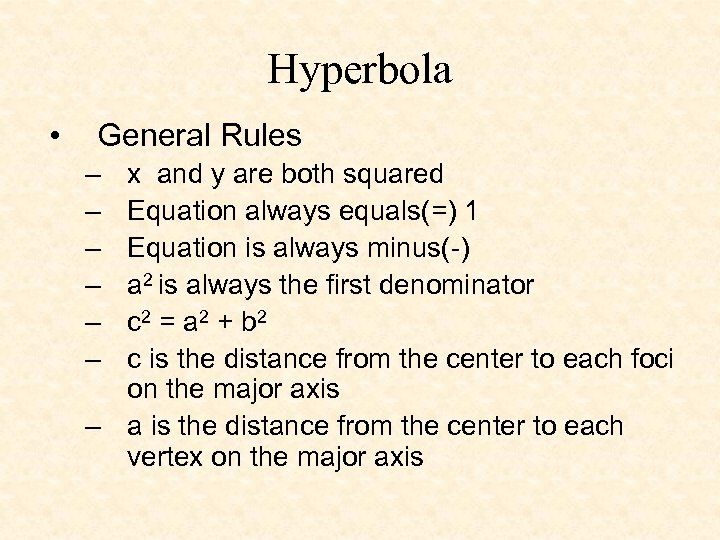Hyperbola • General Rules – – – x and y are both squared Equation always equals(=) 1 Equation is always minus(-) a 2 is always the first denominator c 2 = a 2 + b 2 c is the distance from the center to each foci on the major axis – a is the distance from the center to each vertex on the major axis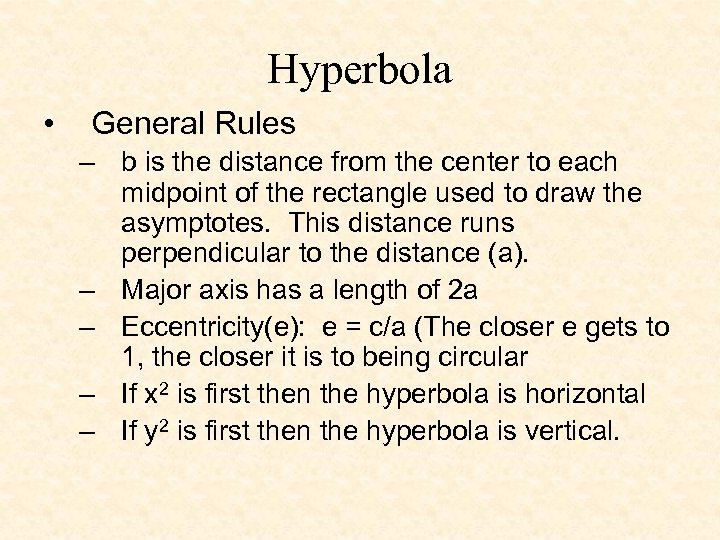Hyperbola • General Rules – b is the distance from the center to each midpoint of the rectangle used to draw the asymptotes. This distance runs perpendicular to the distance (a). – Major axis has a length of 2 a – Eccentricity(e): e = c/a (The closer e gets to 1, the closer it is to being circular – If x 2 is first then the hyperbola is horizontal – If y 2 is first then the hyperbola is vertical.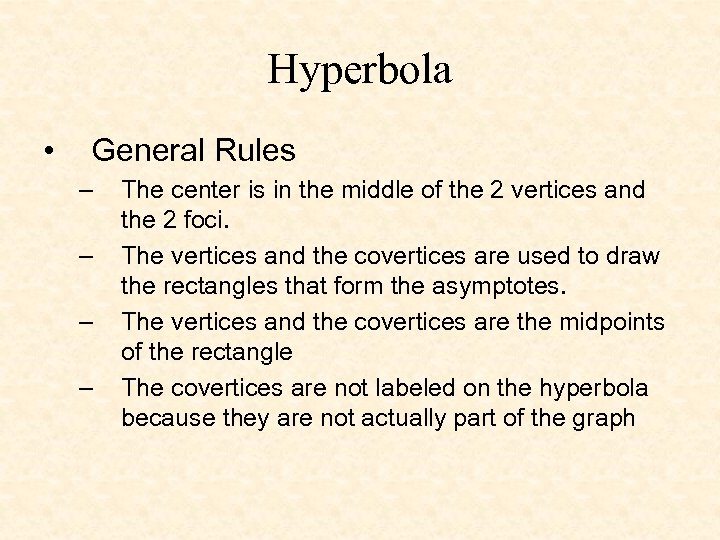Hyperbola • General Rules – – The center is in the middle of the 2 vertices and the 2 foci. The vertices and the covertices are used to draw the rectangles that form the asymptotes. The vertices and the covertices are the midpoints of the rectangle The covertices are not labeled on the hyperbola because they are not actually part of the graph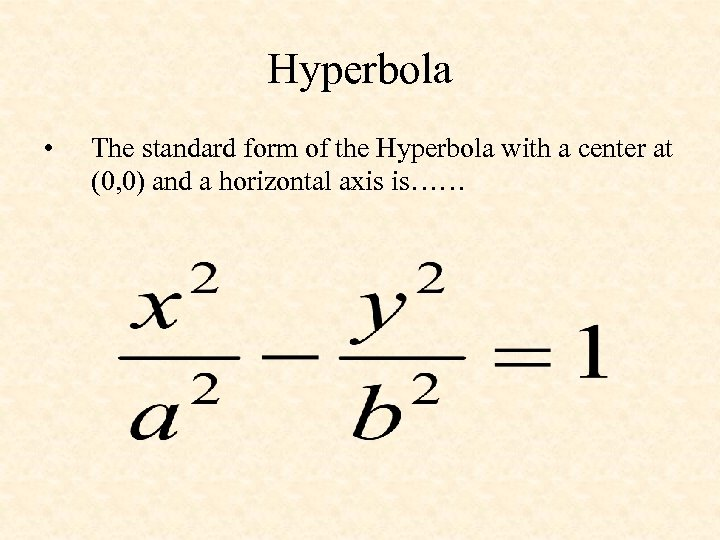Hyperbola • The standard form of the Hyperbola with a center at (0, 0) and a horizontal axis is……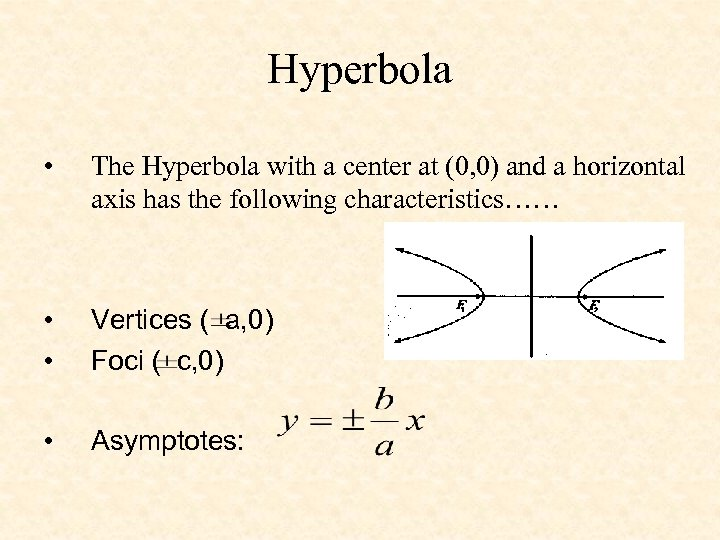Hyperbola • The Hyperbola with a center at (0, 0) and a horizontal axis has the following characteristics…… • • Vertices ( a, 0) Foci ( c, 0) • Asymptotes:Hyperbola • The standard form of the Hyperbola with a center at (0, 0) and a vertical axis is……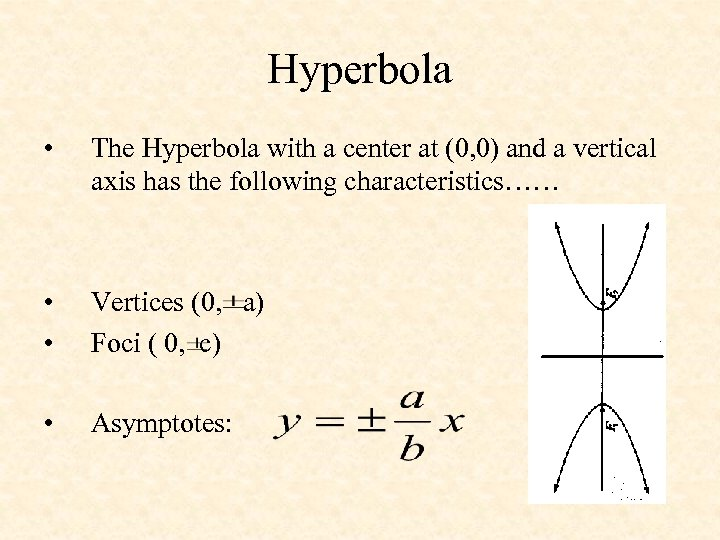Hyperbola • The Hyperbola with a center at (0, 0) and a vertical axis has the following characteristics…… • • Vertices (0, a) Foci ( 0, c) • Asymptotes: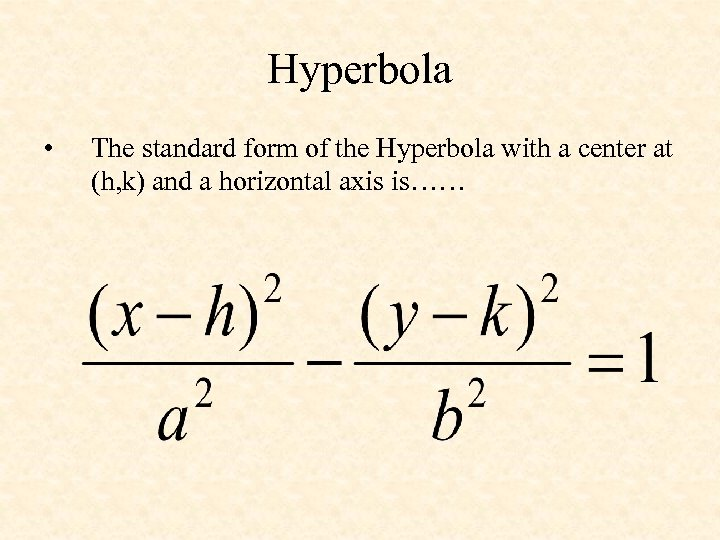Hyperbola • The standard form of the Hyperbola with a center at (h, k) and a horizontal axis is……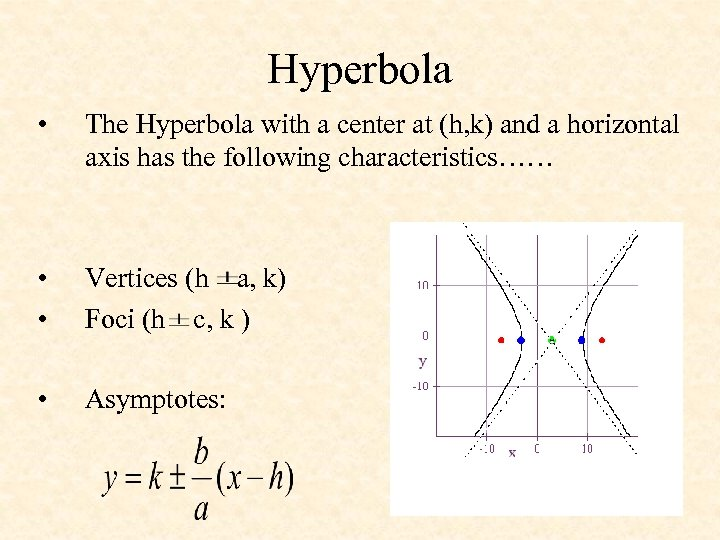Hyperbola • The Hyperbola with a center at (h, k) and a horizontal axis has the following characteristics…… • • Vertices (h a, k) Foci (h c, k ) • Asymptotes: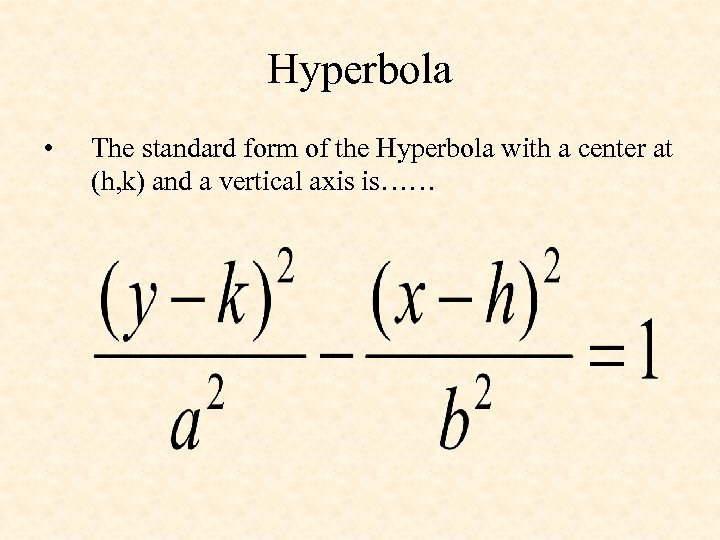Hyperbola • The standard form of the Hyperbola with a center at (h, k) and a vertical axis is……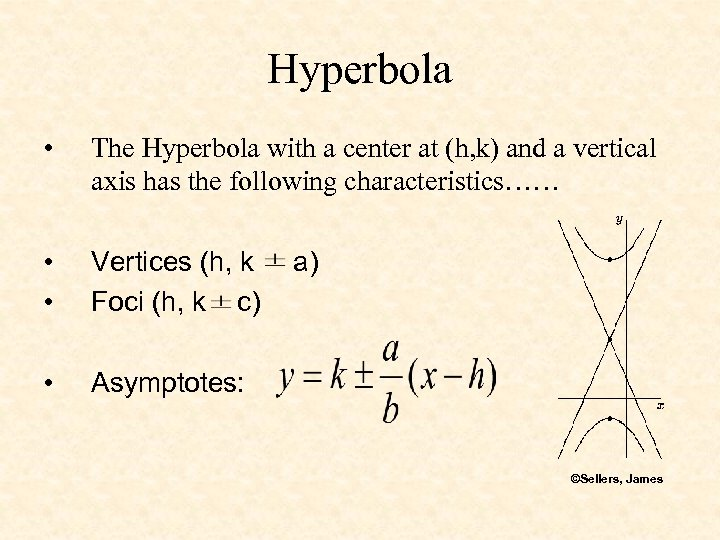Hyperbola • The Hyperbola with a center at (h, k) and a vertical axis has the following characteristics…… • • Vertices (h, k a) Foci (h, k c) • Asymptotes: ©Sellers, James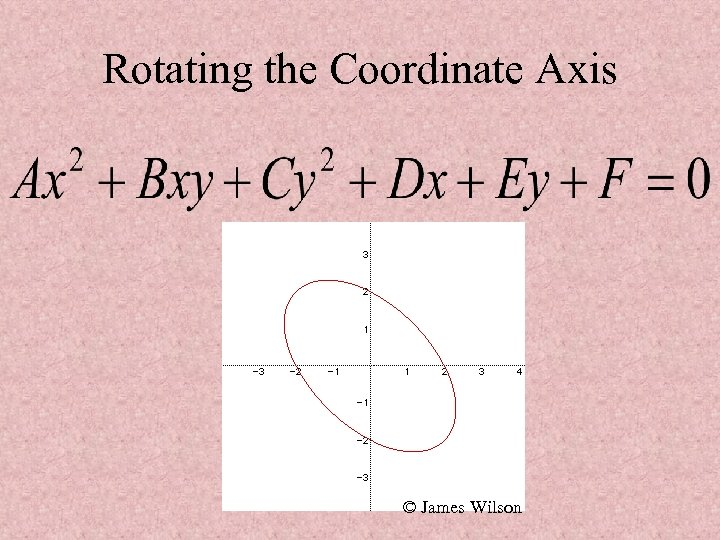Rotating the Coordinate Axis © James Wilson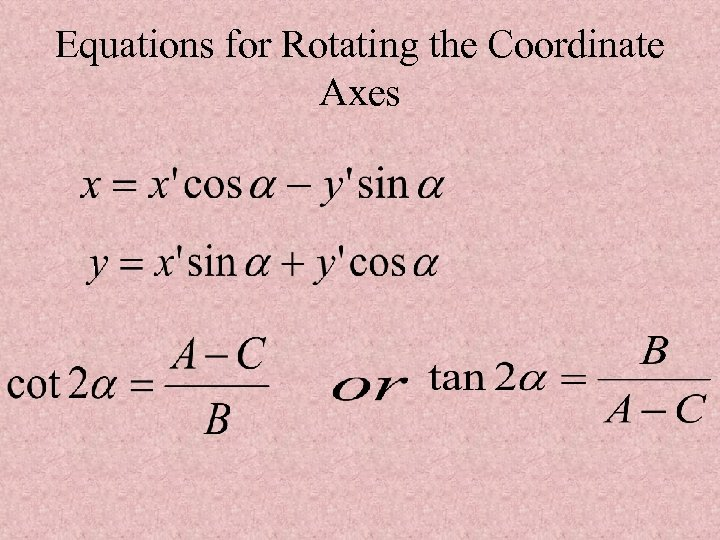Equations for Rotating the Coordinate Axes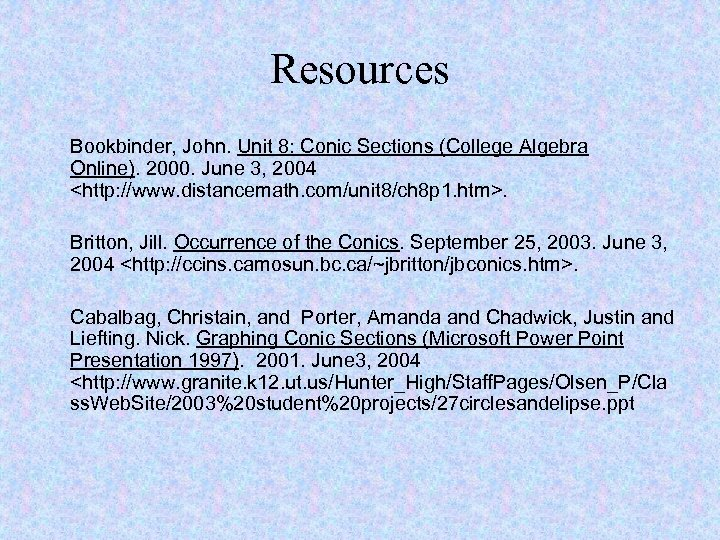Resources Bookbinder, John. Unit 8: Conic Sections (College Algebra Online). 2000. June 3, 2004 . Britton, Jill. Occurrence of the Conics. September 25, 2003. June 3, 2004 . Cabalbag, Christain, and Porter, Amanda and Chadwick, Justin and Liefting. Nick. Graphing Conic Sections (Microsoft Power Point Presentation 1997). 2001. June 3, 2004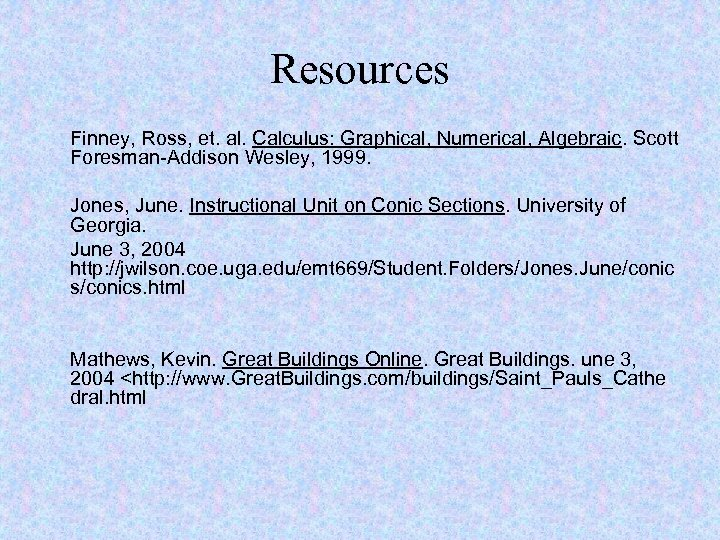Resources Finney, Ross, et. al. Calculus: Graphical, Numerical, Algebraic. Scott Foresman-Addison Wesley, 1999. Jones, June. Instructional Unit on Conic Sections. University of Georgia. June 3, 2004 http: //jwilson. coe. uga. edu/emt 669/Student. Folders/Jones. June/conic s/conics. html Mathews, Kevin. Great Buildings Online. Great Buildings. une 3, 2004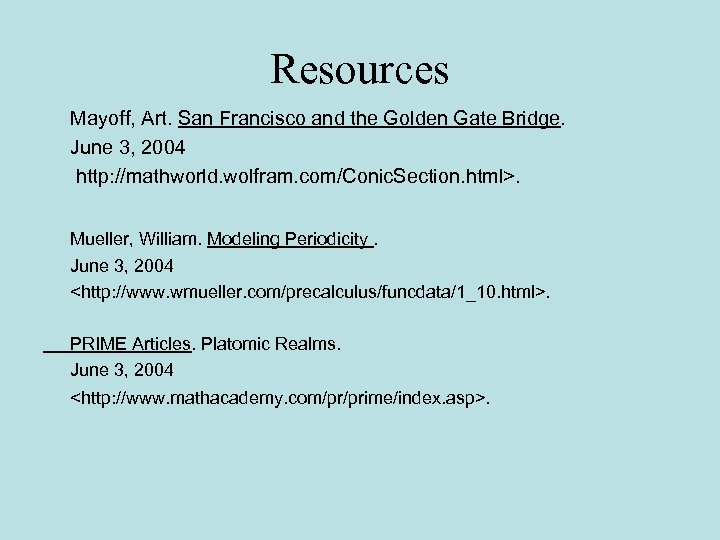Resources Mayoff, Art. San Francisco and the Golden Gate Bridge. June 3, 2004 http: //mathworld. wolfram. com/Conic. Section. html>. Mueller, William. Modeling Periodicity. June 3, 2004 . PRIME Articles. Platomic Realms. June 3, 2004 .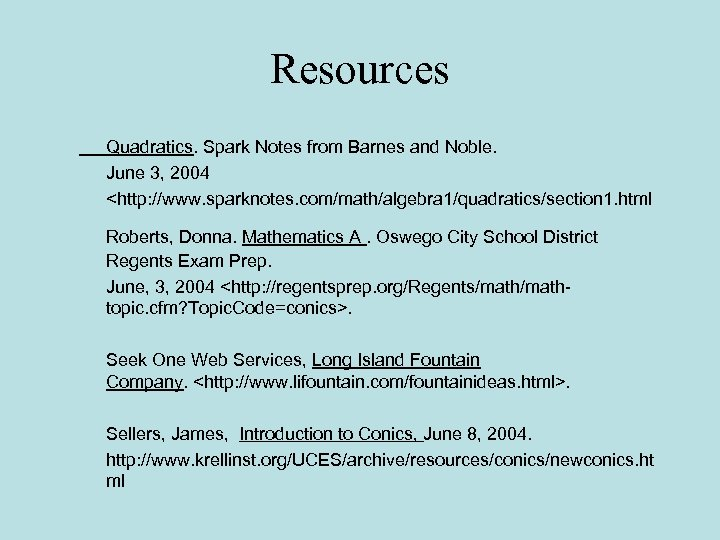Resources Quadratics. Spark Notes from Barnes and Noble. June 3, 2004 . Seek One Web Services, Long Island Fountain Company. . Sellers, James, Introduction to Conics, June 8, 2004. http: //www. krellinst. org/UCES/archive/resources/conics/newconics. ht ml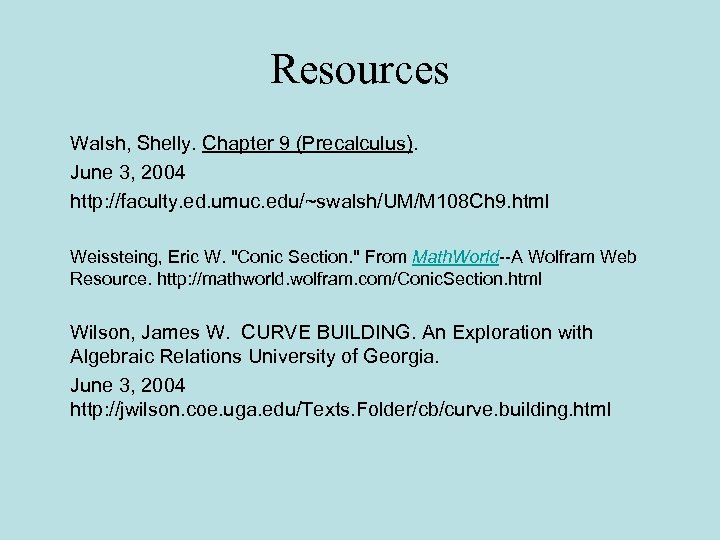Resources Walsh, Shelly. Chapter 9 (Precalculus). June 3, 2004 http: //faculty. ed. umuc. edu/~swalsh/UM/M 108 Ch 9. html Weissteing, Eric W. "Conic Section. " From Math. World--A Wolfram Web Resource. http: //mathworld. wolfram. com/Conic. Section. html Wilson, James W. CURVE BUILDING. An Exploration with Algebraic Relations University of Georgia. June 3, 2004 http: //jwilson. coe. uga. edu/Texts. Folder/cb/curve. building. html